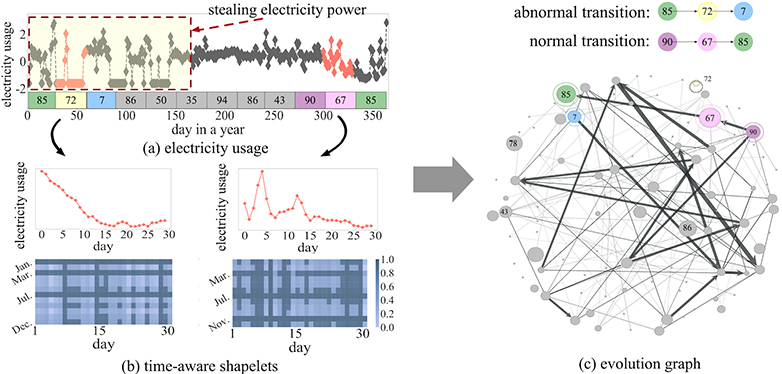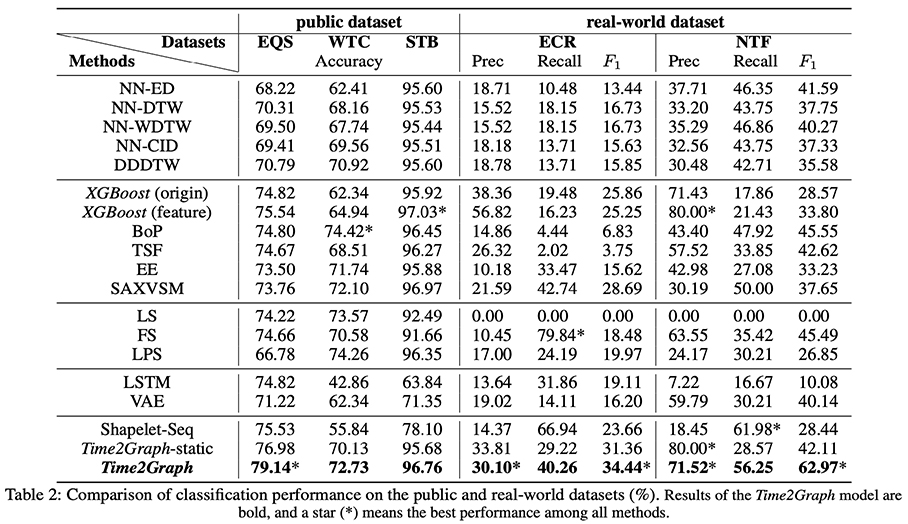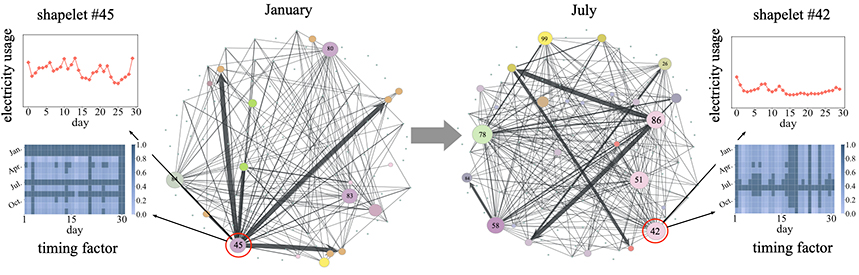View on GitHub

# Time2Graph

## Time2Graph: Revisting Time Series Modeling with Dynamic Shapelets

### Time Series Modeling

Time series modeling aims to discover the temporal relationships within chronologically arranged data. It has attracted extensive research over a wide range of fields, such as image alignment , speech recognition , etc. The key issue here is how to extract the representative features of a time series. A large part of previous frameworks range from classical feature engineering and representation learning to deep learning based models. While these methods have achieved good performance [4, 5], they have also been subject to criticism regarding their lack of interpretability.

### Intuition: Shapelet Dynamics

Shapelets, the time series subsequences that are representative of a class , can offer directly interpretable and explanatory insights in the classification scenario, and shapelet-based models have proven to be promising in various practical domains [7,8,9].

Existing efforts have mainly considered shapelets as static. However, in the real world, shapelets are often dynamic, which is reflected in two respects:

• First, the same shapelet appearing at different time slices may have a range of different impacts. For instance, in the scenario of detecting electricity theft, low electricity consumption in summer or winter is more suspicious than it is in spring, as refrigeration or heating equipments costs more electrical power.
• Second, determining the ways in which shapelets evolve is vital to a full understanding of a time series. In fact, shapelets with small values at a particular time can hardly distinguish an electricity thief from a normal user who indeed consumes a low level of electricity. An alternative method would involve identifying users who once had high electricity consumption shapelets but suddenly consumes very few electrical power. In other words, an important clue here is how shapelets evolve over time.

We refer to the subsequences of a time series that are able to reflect its representativeness at different time slices as time-aware shapelets. Furthermore, to deeply mining the dynamics and correlations of shapelets, we propose a novel approach to learn the representations of a time series by extracting time-aware shapelets and constructing a shapelet evolution graph, referred as our AAAI’2020 paper .Above shows an concrete example from real-world electricity consumption record data, which may better explain our motivations: Fig. a demonstrates the one-year electricity usage of a user who has stolen electrical power from January to May while using electrical power normally in the remaining months. We assign each month the most representative shapelet at that time and present the shapelets #72 and #67, along with their timing factors in Fig. b, where dark areas indicate that the corresponding shapelet is more discriminative relative to light areas. The shapelet evolution graph is presented in Fig. c, illustrating how a shapelet would transfer from one to another in a normal case: for the normal electricity consumption record, there is a clear path for its shapelet transition (#90#67#85) in the graph. For the abnormal data, however, the path (#85#72#7) does not exist, indicating that the connectivity of the shapelet transition path provides an evidential basis for detecting an abnormal time series. Finally, we translate the problem of learning representations of shapelets and time series into a graph embedding problem.

### Extracting Time-aware Shapelets

Formally, a shapelet $v$ is a segment that is representative of a certain class. More precisely, it can separate $T$ into two smaller sets, one that is close to $v$ and another far from $v$ by some specific criteria, such that for a time series classification task, positive and negative samples can be put into different groups. The criteria can be formalized as

where $S_{*}(v, T)$ denotes the set of distances with respect to a specific group $T_{*}$, and the function $g$ takes two finite sets as input, returns a scalar value to indicate how far these two sets are, and it can be information gain, or some dissimilarity measurements on sets, i.e., KL divergence.

To capture the shapelet dynamics, We define two factors for quantitatively measuring the timing effects of shapelets at different levels. Specifically, we introduce the local factor $w_n$​ to denote the inner importance of the n-th element of a particular shapelet, then the distance between a shapelet $v$ and a segment $s$ is redefined as

where $a^*$ refers to the best alignment for DTW distance. On the other hand, at a global level, we aim to measure the timing effects across segments on the discriminatory power of shapelets. It is inspired from the intuition that shapelets may represent totally different meaning at different time steps, and it is straightforward to measure such deviations by adding segment-level weights. Formally, we set a global factor $u_m$ to capture the cross-segments influence, then the distance between a shapelet $v$ and a time series $t$ can be rewritten as

Then given a classification task, we establish a supervised learning method to select the most important time-aware shapelets and learn corresponding timing factors $w_i$ and $u_i$ for each shapelet $v_i$. In particular, we have a pool of segments as shapelet candidates that selected from all subsequences, and a set of time series $T$ with labels. For each candidate $v$, we have the following objective function:

and after learning the timing factors from shapelet candidates separately, we select the top K shapelets with minimal loss as our final time-aware shapelets.

### Constructing Shapelet Evolution Graph

A Shapelet Evolution Graph is a directed and weighted graph $G = (V,E)$ in which $V$ consists of $K$ vertices, each denoting a shapelet, and each directed edge $e_{i, j} \in E$ is associated with a weight $w_{i, j}$, indicating the occurrence probability of shapelet $v_i \in V$ followed by another shapelet $v_j \in V$ in the same time series. The key idea here is that the shapelet evolution and transition patterns can be naturally reflected from the paths in the graph, then graph embedding mythologies can be applied to learn shapelet, as well as the time series representations.

We first assign each segment $s_i$ of each time series to several shapelets that have the closest distances to $s_i$ according to the time-aware dissimilarity. In detail, we standardize the shapelet assignment probability as

where

with a predefined constraint that $\hat{d_{i, *}} \le \delta$. Then, for each pair $(j, k)$, we create a weighted edge from shapelet $v_{i, j}$ to $v_{i+1, k}$ with weight $p_{i, j} \cdot p_{i+1, k}$ , and merge all duplicated edges as one by summing up their weights. Finally, we normalize the edge weights sourced from each node as 1, which naturally interprets the edge weight between each pair of nodes, i.e., $v_i$ and $v_j$ into the conditional probability that shapelet $v_i$ being transformed into $v_j$ in an adjacent time step.

### Time Series Representation Learning

Finally, we learn the representations for both the shapelets and the given time series by using the shapelet evolution graph constructed as above. We first employ an existing graph embedding algorithm DeepWalk  to obtain vertex (shapelet) representation vectors $\mu \in \mathbb{R}^B$. Then, for each segment $s_i$ in a time series, we retrieve the embeddings of its assigned shapelets that have discussed above, and sum them up weighted by assignment probability, denoted as

and finally concatenate or aggregate all those $m$ segment embedding vectors to obtain the representation vector for original time series $t$. The time series embeddings can then be applied to various down streaming tasks, referred to the experiment section in our paper .

### Evaluation Results

We conduct time series classification tasks on three public benchmarks datasets from UCR-Archive  and two real-world datasets from State Grid of China and China Telecom. Experimental results are shown in the following table:We have also conduct extensive ablation and observational studies to validate our proposed framework. Here we construct the shapelet evolution graphs at different time steps for deeper understanding of shapelet dynamics, seen in the figure below. It shows two graphs, one for January and another for July. In January, shapelet #45 has large in/out degrees, and its corresponding timing factor is highlighted in January and February (dark areas). It indicates that shapelet #45 is likely to be a common pattern at the beginning of a year. As for July, shapelet #45 is no longer as important as it was in January. Meanwhile, shapelet #42, which is almost an isolated point in January, becomes very important in July. Although we do not explicitly take seasonal information into consideration when constructing shapelet evolution graphs, the inclusion of the timing factors means that they are already incorporated into the process of the graph generation.### Reference

 Cheng, Z; Yang, Y; Wang, W; Hu, W; Zhuang, Y and Song, G, 2020, Time2Graph: Revisiting Time Series Modeling with Dynamic Shapelets, In AAAI, 2020

 Peng, X.; Huang, J.; Hu, Q.; Zhang, S.; and Metaxas, D. N. 2014. Head pose estimation by instance parameterization. In ICPR’14, 1800–1805.

 Shimodaira, H.; Noma, K.-i.; Nakai, M.; and Sagayama, S. 2002. Dynamic time-alignment kernel in support vector machine. In NIPS’02, 921–928.

 Malhotra, P.; Ramakrishnan, A.; Anand, G.; Vig, L.; Agar- wal, P.; and Shroff, G. 2016. Lstm-based encoder- decoder for multi-sensor anomaly detection. arXiv preprint arXiv:1607.00148.

 Johnson, M.; Duvenaud, D. K.; Wiltschko, A.; Adams, R. P.; and Datta, S. R. 2016. Composing graphical models with neu- ral networks for structured representations and fast inference. In NIPS’16, 2946–2954.

 Ye, L., and Keogh, E. 2011. Time series shapelets: a novel technique that allows accurate, interpretable and fast classifi- cation. DMKD. 22(1):149–182.

 Bostrom, A., and Bagnall, A. 2017. Binary shapelet trans- form for multiclass time series classification. In TLSD- KCS’17. 24–46.

 Hills, J.; Lines, J.; Baranauskas, E.; Mapp, J.; and Bagnall, A. 2014. Classification of time series by shapelet transformation. DMKD. 28(4):851–881

 Lines, J.; Davis, L. M.; Hills, J.; and Bagnall, A. 2012. A shapelet transform for time series classification. In KDD’12, 289–297.

 Perozzi, B.; Al-Rfou, R.; and Skiena, S. 2014. Deepwalk: Online learning of social representations. In KDD, 701–710.

 Dau, H. A.; Keogh, E.; Kamgar, K.; Yeh, C.-C. M.; Zhu, Y.; Gharghabi, S.; Ratanamahatana, C. A.; Yanping; Hu, B.; Begum, N.; Bagnall, A.; Mueen, A.; and Batista, G. 2018. The ucr time series classification archive. https://www.cs.ucr.edu/~eamonn/time_series_data_2018/.